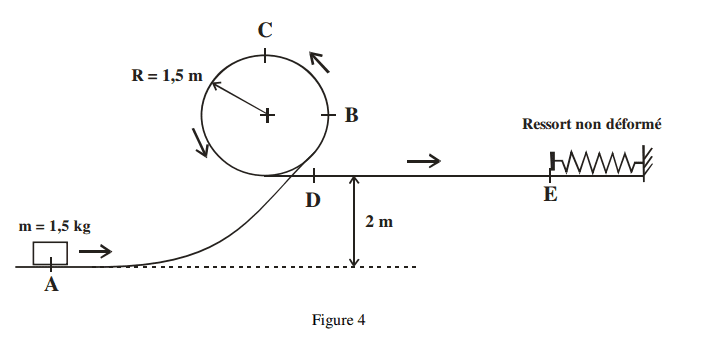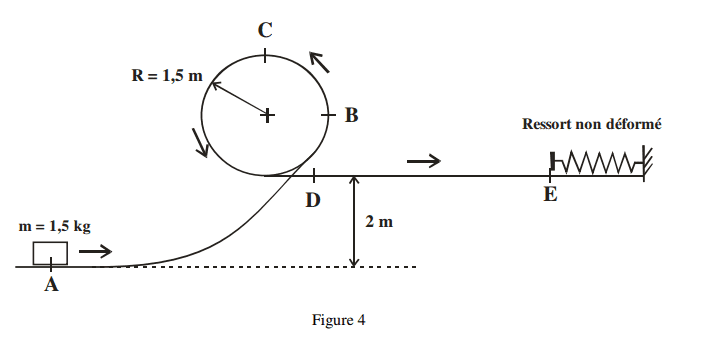# Block and spring on a weird track (roller coaster)

## Homework Statement

A block of mass m = 1.5 kg is launched from point A with speed VA = 12 m / s, in the circuit
shown in Figure 4. If we assume that there is no friction determine:
a) the speed of the block when it reaches the point C;

Drawing of the problem:
http://i.imgur.com/TrcMJ5I.png

## Homework Equations

This question has about 10 questions but I only want to really understand the first one and I would like your help with this because its very confusing me since the ramp is curved and not straight plane like usual.
Also the circular part is confusing me a lot too.

## The Attempt at a Solution

Do I have to use the conservative energy formula to find the final speed to C ?

I am very sorry I dont have anything as my own work (try) but I really do not know how to do this.
I have never posted a help thread before without any work done.

SammyS
Staff Emeritus
Homework Helper
Gold Member

## Homework Statement

A block of mass m = 1.5 kg is launched from point A with speed VA = 12 m / s, in the circuit
shown in Figure 4. If we assume that there is no friction determine:
a) the speed of the block when it reaches the point C;

Drawing of the problem:
http://i.imgur.com/TrcMJ5I.png

## Homework Equations

This question has about 10 questions but I only want to really understand the first one and I would like your help with this because its very confusing me since the ramp is curved and not straight plane like usual.
Also the circular part is confusing me a lot too.

## The Attempt at a Solution

Do I have to use the conservative energy formula to find the final speed to C ?

I am very sorry I don't have anything as my own work (try) but I really do not know how to do this.
I have never posted a help thread before without any work done.What is the initial Kinetic Energy of the block?

What is the change in potential energy of the block in going from point A to point C ?What is the initial Kinetic Energy of the block?

What is the change in potential energy of the block in going from point A to point C ?
Ek = 1/2*m*vi2
Ek = 1/2*1.5kg*12m/s
Ek = 9J

Ugf-Ugi = m*g*hf - m*g*hi
(1.5kg*9.81*0) - 1.5kg*9.81*(2m+1.5m)
= -51.5025J

SammyS
Staff Emeritus
Homework Helper
Gold Member
Ek = 1/2*m*vi2
Ek = 1/2*1.5kg*12m/s
Ek = 9J

Ugf-Ugi = m*g*hf - m*g*hi
(1.5kg*9.81*0) - 1.5kg*9.81*(2m+1.5m)
= -51.5025J
You didn't square the velocity for kinetic energy.

You have the potential energies reversed. UC - UA is positive .

You didn't square the velocity for kinetic energy.

You have the potential energies reversed. UC - UA is positive .
Ek= 108J
and potential energy is 51.5025J

Then I use this formula to find the Vf?
Ugi+1/2*m*vi2 = Ugf + 1/2*m*vf2
108J = 51.5025J+ 1/2*1.5Kg*vf2
vf = 8.67929m/s

You didn't square the velocity for kinetic energy.

You have the potential energies reversed. UC - UA is positive .
Then
b) the centripetal acceleration ( normal) in point C;
I would simply do this?
ac = v2/R
ac = 8.679292/1.5
=50.22m/s2

c) the normal reaction exerted by the loop on the block at point C (Draw the diagram of the forces and acceleration) ;
Do not really understand this.

Would N be the same direction as W in this situation since the block is under the plane.
Forces in Y = 0 = - N - W
= 0 = - N - 1.5*9.81
N = -14.715N

SammyS
Staff Emeritus
Homework Helper
Gold Member
Would N be the same direction as W in this situation since the block is under the plan
Yes, but the following is not correct. The acceleration is not zero. See part (b).
Forces in Y = 0 = - N - W
= 0 = - N - 1.5*9.81
N = -14.715N
You assumed the normal force was downward, but the result came out negative, which would make the normal force be upward.

Yes, but the following is not correct. The acceleration is not zero. See part (b).

You assumed the normal force was downward, but the result came out negative, which would make the normal force be upward.
Then the gravity is upward as well?
Yes, but the following is not correct. The acceleration is not zero. See part (b).

You assumed the normal force was downward, but the result came out negative, which would make the normal force be upward.
http://i.imgur.com/jzuoGuC.png is my diagram of forces and acceleration correct?

If that is correct then I can do this:
Forces in Y = - m*ac = - N - W
Forces in Y = - (1.5kg*50.22m/s2c) = - N - (1.5kg*9,81)
now my N is 60.615N

is that correct?

Thanks for helping me, I really appreciate it :)

SammyS
Staff Emeritus
Homework Helper
Gold Member
In reply to a comment of mine you wrote:
Then the gravity is upward as well?

No. Gravity is downward. The fact that N turns out to be upward came from you ignoring centripetal acceleration. If the block were to remain stationary on the bottom side of the track at point C, then the track would have to exert a force upward to compensate for gravity.
http://i.imgur.com/jzuoGuC.png is my diagram of forces and acceleration correct?

If that is correct then I can do this:
Forces in Y = - m*ac = - N - W
Forces in Y = - (1.5kg*50.22m/s2c) = - N - (1.5kg*9,81)
now my N is 60.615N

is that correct?

Thanks for helping me, I really appreciate it :)
That is a correct method. Looks good.

In reply to a comment of mine you wrote:

No. Gravity is downward. The fact that N turns out to be upward came from you ignoring centripetal acceleration. If the block were to remain stationary on the bottom side of the track at point C, then the track would have to exert a force upward to compensate for gravity.

That is a correct method. Looks good.
Now to find :
d) the speed of the block as it passes under D and E;

For D:

Ugi = m*g*hi = 1.5kg*9.81*(2+1.5) = 51.5025J
Ugf = m*g*hf = 1.5kg*9.81*(2) = 29.43J
Eki = 1/2*m*vi2 = 1/2*1.5kg*8.679292 =56.4976J
Ekf = 1/2*m*vf2 = 1/2*1.5kg*vf2

Ugi + Eki = Ugf +Ekf
51.5025J + 56.4976J= 29.43J + 1/2*1.5kg*vf2
vf = 10.2352m/s ---> D

and now for E;

Ugi = m*g*hi = 1.5kg*9.81*(2) = 29.43J
Ugf = m*g*hf = 1.5kg*9.81*(2) = 29.43J
Eki = 1/2*m*vi2 = 1/2*1.5kg*10.2352m/s2 =78.5695J
Ekf = 1/2*m*vf2 = 1/2*1.5kg*vf2

Ugf - Ugi = 0
Eki = Ekf
78.5695J = 1/2*1.5kg*vf2
= 10.2342m/s

normal that my speed is the same ? there is no acceleration I should add somewhere :/?

thanks.